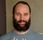cancel
Showing results for
Did you mean:Anonymous
Not applicable

## Filtering a cloumn with text

Hi

Pleas can someone help with this.  I have searched and cant find the answer to my question(s).

How to filter a column with text only without using the page or visual filters.

I am creating a report that shows how many sales have been made by source.  We have many online platforms and this leads me onto my next question.

What i was hoping to do was to filter by source.  Then each of the sources has their own fees to take into account.  Over all i am wanting to be able to create a report that does the following.

Calculates totals by source.

deduct the differnt fees per source to provide an exact profit report.

Exaple

Source 1, base fee 0.30p per transaction, 12% of total order.

Source 2, base fee 0.30p per transaction, 5% of total order.

Source 3, base fee 0.30p per transaction, 7% of total order.

Source 4, base fee 0.00p per transaction, 20% of total order.

Source 5, base fee,  15% of total order. or £1 depending what value is more

I have a calender table and date table. with most of the measures as i am 80 %complete just need this part answering.

Many thanks

Ben

1 ACCEPTED SOLUTIONSuper User

@Anonymous ,

since you have provided very little information about your model and data, this is a bit of guess work. Are you sure you can't handle fees within a calculated column, like this:

``````sales fee =
VAR _sumFees =
transactions[total order value]
* LOOKUPVALUE (
dimFees[base transaction fee];
dimFees[source]; transactions[source]
)
+ transactions[total order value]
* LOOKUPVALUE (
dimFees[percentage of total order];
dimFees[source]; transactions[source]
)
VAR _minFee =
LOOKUPVALUE ( dimFees[minimum value]; dimFees[source]; transactions[source] )
RETURN
IF ( _sumFees > _minFee; _sumFees; _minFee )``````

if you need it as a measure you should look into the sumx-function

``````Measure =
SUMX (
VALUES ( transactions[transactionID] );
VAR _source =
SELECTEDVALUE ( transactions[source] )
VAR _minFee =
LOOKUPVALUE ( dimFees[minimum value]; dimFees[source]; _source )
VAR _baseFeePrTrans =
LOOKUPVALUE ( dimFees[base transaction fee]; dimFees[source]; _source )
VAR _totalFee =
LOOKUPVALUE ( dimFees[percentage of total order]; dimFees[source]; _source )
RETURN
IF (
CALCULATE ( SUM ( transactions[total order value] ) ) * _totalFee
+ CALCULATE ( COUNT ( transactions[transactionID] ) ) * _baseFeePrTrans < _minFee;
_minFee;
CALCULATE ( SUM ( transactions[total order value] ) ) * _totalFee
+ CALCULATE ( COUNT ( transactions[transactionID] ) ) * _baseFeePrTrans
)
)``````

Cheers,
Sturla

If this post helps, then please consider Accepting it as the solution. Kudos are nice too.Super User

@Anonymous ,

since you have provided very little information about your model and data, this is a bit of guess work. Are you sure you can't handle fees within a calculated column, like this:

``````sales fee =
VAR _sumFees =
transactions[total order value]
* LOOKUPVALUE (
dimFees[base transaction fee];
dimFees[source]; transactions[source]
)
+ transactions[total order value]
* LOOKUPVALUE (
dimFees[percentage of total order];
dimFees[source]; transactions[source]
)
VAR _minFee =
LOOKUPVALUE ( dimFees[minimum value]; dimFees[source]; transactions[source] )
RETURN
IF ( _sumFees > _minFee; _sumFees; _minFee )``````

if you need it as a measure you should look into the sumx-function

``````Measure =
SUMX (
VALUES ( transactions[transactionID] );
VAR _source =
SELECTEDVALUE ( transactions[source] )
VAR _minFee =
LOOKUPVALUE ( dimFees[minimum value]; dimFees[source]; _source )
VAR _baseFeePrTrans =
LOOKUPVALUE ( dimFees[base transaction fee]; dimFees[source]; _source )
VAR _totalFee =
LOOKUPVALUE ( dimFees[percentage of total order]; dimFees[source]; _source )
RETURN
IF (
CALCULATE ( SUM ( transactions[total order value] ) ) * _totalFee
+ CALCULATE ( COUNT ( transactions[transactionID] ) ) * _baseFeePrTrans < _minFee;
_minFee;
CALCULATE ( SUM ( transactions[total order value] ) ) * _totalFee
+ CALCULATE ( COUNT ( transactions[transactionID] ) ) * _baseFeePrTrans
)
)``````

Cheers,
Sturla

If this post helps, then please consider Accepting it as the solution. Kudos are nice too.Announcements#### Exclusive opportunity for Women!

Join us for a free, hands-on Microsoft workshop led by women trainers for women where you will learn how to build a Dashboard in a Day!#### Power Platform Conference-Power BI and Fabric Sessions

Join us Oct 1 - 6 in Las Vegas for the Microsoft Power Platform Conference.Top Solution Authors
Top Kudoed Authors
Users online (1,927)